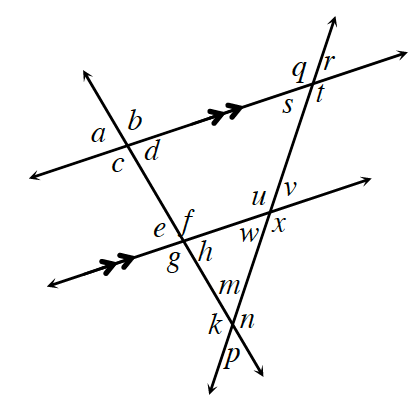### Home > CCG > Chapter 11 > Lesson 11.1.4 > Problem11-64

11-64.Examine the diagram at right. State the relationship between each pair of angles listed below (such as vertical angles) and state whether the angles are congruent, supplementary, or neither.

See the Math Notes box in Lesson 2.1.4 if you need to review angle relationships.

1. $∠e$ and $∠a$

corresponding angles, $≅$

2. $∠t$ and $∠u$

alternate interior angles, $≅$

3. $∠v$ and $∠x$

4. $∠g$ and $∠v$